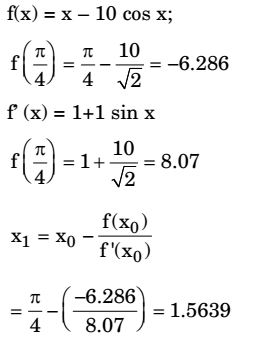## Engineering Mathematics Miscellaneous

#### Engineering Mathematics

1. The root of the function f(x) = x3 + x – 1 obtained after first iteration on application of Newton Raphson scheme using an initial guess of x0 = 1 is

1. f (x) = x3 + x–1
f (1) = 1+1 – 1 = 1
f’(x) = 3x2 + 1
f’ (1) = 3+1=4

 x1 = x0 - f(x0) = 1 - 1 f'(x0) 4

= 1 – 0.25
x1 = 0.75

##### Correct Option: C

f (x) = x3 + x–1
f (1) = 1+1 – 1 = 1
f’(x) = 3x2 + 1
f’ (1) = 3+1=4

 x1 = x0 - f(x0) = 1 - 1 f'(x0) 4

= 1 – 0.25
x1 = 0.75

1. Solve the equation x = 10 cos(x) using the Newton-Raphson method. The initial guess is x = π / 4. The value of the predicted root after the first iteration, up to second decimal, is _____.

1.##### Correct Option: A1. Newton-Raphson method is used to find the roots of the equation, x3 + 2x2 + 3x – 1 = 0. If the initial guess is x0 = 1, then the value of x after 2nd iteration is ________.

1. By Newton-Raphson Method,

 1st iteration, x1 = x0 – f(0) f'(0)

 = 1 - f(1) = 1 - 5 = 1 f'(1) 10 2

Where f(x) = x3 + 2x2 + 3x – 1 ⇒ f(1) = 5
f'(x) = 3x2 + 4x + 3 ⇒ f'(1) = 10
 2nd iteration, x2 = x1 f(x1) = 0.5 - f(0.5) = 0.3043 f'(x1) f'(0.5)

##### Correct Option: A

By Newton-Raphson Method,

 1st iteration, x1 = x0 – f(0) f'(0)

 = 1 - f(1) = 1 - 5 = 1 f'(1) 10 2

Where f(x) = x3 + 2x2 + 3x – 1 ⇒ f(1) = 5
f'(x) = 3x2 + 4x + 3 ⇒ f'(1) = 10
 2nd iteration, x2 = x1 f(x1) = 0.5 - f(0.5) = 0.3043 f'(x1) f'(0.5)

1. The real root of the equation 5x – 2 cosx – 1 = 0 (up to two decimal accuracy) is _______.

1. Let f(x) =5x – 2 cos x – 1
⇒ f'(x) = 5 + 2 sin x
f(0) = – 3; f(1) = 2.9
By intermediate value theorem roots lie between 0 and 1.
Let x0 = 1 rad = 57.32º
By Newton Raphson method,

 Xn+1 = Xn - f(xn) f'(xn)

 ⇒ xn+1 2xnsin xn + 2 cos xn + 1 5 + 2Sin xn

⇒ x1 = 0.5632
⇒ x2 = 0.5425
⇒ x3 = 0.5424

##### Correct Option: A

Let f(x) =5x – 2 cos x – 1
⇒ f'(x) = 5 + 2 sin x
f(0) = – 3; f(1) = 2.9
By intermediate value theorem roots lie between 0 and 1.
Let x0 = 1 rad = 57.32º
By Newton Raphson method,

 Xn+1 = Xn - f(xn) f'(xn)

 ⇒ xn+1 2xnsin xn + 2 cos xn + 1 5 + 2Sin xn

⇒ x1 = 0.5632
⇒ x2 = 0.5425
⇒ x3 = 0.5424

1. Let X and Y be two independent random variables. Which one of the relations between expectation (n), variance (Var) and covariance (Cov) given below is FALSE?

1. X and Y are independent
∴ (a), (b), (c) are true
Only (d) is odd one

##### Correct Option: D

X and Y are independent
∴ (a), (b), (c) are true
Only (d) is odd one